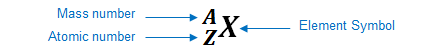# Problem: An atom has a mass number of 27 and 14 neutrons. what is the atomic number of this atom?

###### FREE Expert Solution

We’re being asked to determine the atomic number of an atom that has a mass number of 27 and 14 neutrons

Recall: an element can be represented as:You can determine the identity of the element using its atomic number:

Atomic number (Z):

82% (348 ratings)###### Problem Details

An atom has a mass number of 27 and 14 neutrons. what is the atomic number of this atom?